Vous devez avoir un compte Developpez.com et être connecté pour pouvoir participer aux discussions.

##### Identifiez-vous
 Identifiant Mot de passe Mot de passe oublié ?
##### Créer un compte

Vous n'avez pas encore de compte Developpez.com ? L'inscription est gratuite et ne vous prendra que quelques instants !Developpez.com

Python

Choisissez la catégorie, puis la rubrique :

7.4. Using the {n,m} Syntax

## 7.4. Using the {n,m} Syntax

In the previous section, you were dealing with a pattern where the same character could be repeated up to three times. There is another way to express this in regular expressions, which some people find more readable. First look at the method we already used in the previous example.

### Example 7.5. The Old Way: Every Character Optional

```>>> import re
>>> pattern = '^M?M?M?\$'
>>> re.search(pattern, 'M')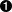<_sre.SRE_Match object at 0x008EE090>
>>> pattern = '^M?M?M?\$'
>>> re.search(pattern, 'MM')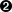<_sre.SRE_Match object at 0x008EEB48>
>>> pattern = '^M?M?M?\$'
>>> re.search(pattern, 'MMM')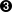<_sre.SRE_Match object at 0x008EE090>
>>> re.search(pattern, 'MMMM')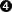>>>
```This matches the start of the string, and then the first optional M, but not the second and third M (but that's okay because they're optional), and then the end of the string.This matches the start of the string, and then the first and second optional M, but not the third M (but that's okay because it's optional), and then the end of the string.This matches the start of the string, and then all three optional M, and then the end of the string.This matches the start of the string, and then all three optional M, but then does not match the the end of the string (because there is still one unmatched M), so the pattern does not match and returns None.

### Example 7.6. The New Way: From n o m

```>>> pattern = '^M{0,3}\$'>>> re.search(pattern, 'M')<_sre.SRE_Match object at 0x008EEB48>
>>> re.search(pattern, 'MM')<_sre.SRE_Match object at 0x008EE090>
>>> re.search(pattern, 'MMM')<_sre.SRE_Match object at 0x008EEDA8>
>>> re.search(pattern, 'MMMM')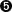>>>
```This pattern says: “Match the start of the string, then anywhere from zero to three M characters, then the end of the string.” The 0 and 3 can be any numbers; if you want to match at least one but no more than three M characters, you could say M{1,3}.This matches the start of the string, then one M out of a possible three, then the end of the string.This matches the start of the string, then two M out of a possible three, then the end of the string.This matches the start of the string, then three M out of a possible three, then the end of the string.This matches the start of the string, then three M out of a possible three, but then does not match the end of the string. The regular expression allows for up to only three M characters before the end of the string, but you have four, so the pattern does not match and returns None.There is no way to programmatically determine that two regular expressions are equivalent. The best you can do is write a lot of test cases to make sure they behave the same way on all relevant inputs. You'll talk more about writing test cases later in this book.

### 7.4.1. Checking for Tens and Ones

Now let's expand the Roman numeral regular expression to cover the tens and ones place. This example shows the check for tens.

### Example 7.7. Checking for Tens

```>>> pattern = '^M?M?M?M?(CM|CD|D?C?C?C?)(XC|XL|L?X?X?X?)\$'
>>> re.search(pattern, 'MCMXL')<_sre.SRE_Match object at 0x008EEB48>
>>> re.search(pattern, 'MCML')<_sre.SRE_Match object at 0x008EEB48>
>>> re.search(pattern, 'MCMLX')<_sre.SRE_Match object at 0x008EEB48>
>>> re.search(pattern, 'MCMLXXX')<_sre.SRE_Match object at 0x008EEB48>
>>> re.search(pattern, 'MCMLXXXX')>>>
```This matches the start of the string, then the first optional M, then CM, then XL, then the end of the string. Remember, the (A|B|C) syntax means “match exactly one of A, B, or C”. You match XL, so you ignore the XC and L?X?X?X? choices, and then move on to the end of the string. MCML is the Roman numeral representation of 1940.This matches the start of the string, then the first optional M, then CM, then L?X?X?X?. Of the L?X?X?X?, it matches the L and skips all three optional X characters. Then you move to the end of the string. MCML is the Roman numeral representation of 1950.This matches the start of the string, then the first optional M, then CM, then the optional L and the first optional X, skips the second and third optional X, then the end of the string. MCMLX is the Roman numeral representation of 1960.This matches the start of the string, then the first optional M, then CM, then the optional L and all three optional X characters, then the end of the string. MCMLXXX is the Roman numeral representation of 1980.This matches the start of the string, then the first optional M, then CM, then the optional L and all three optional X characters, then fails to match the end of the string because there is still one more X unaccounted for. So the entire pattern fails to match, and returns None. MCMLXXXX is not a valid Roman numeral.

The expression for the ones place follows the same pattern. I'll spare you the details and show you the end result.

```>>> pattern = '^M?M?M?M?(CM|CD|D?C?C?C?)(XC|XL|L?X?X?X?)(IX|IV|V?I?I?I?)\$'
```

So what does that look like using this alternate {n,m} syntax? This example shows the new syntax.

### Example 7.8. Validating Roman Numerals with {n,m}

```>>> pattern = '^M{0,4}(CM|CD|D?C{0,3})(XC|XL|L?X{0,3})(IX|IV|V?I{0,3})\$'
>>> re.search(pattern, 'MDLV')<_sre.SRE_Match object at 0x008EEB48>
>>> re.search(pattern, 'MMDCLXVI')<_sre.SRE_Match object at 0x008EEB48>
>>> re.search(pattern, 'MMMMDCCCLXXXVIII')<_sre.SRE_Match object at 0x008EEB48>
>>> re.search(pattern, 'I')<_sre.SRE_Match object at 0x008EEB48>
```This matches the start of the string, then one of a possible four M characters, then D?C{0,3}. Of that, it matches the optional D and zero of three possible C characters. Moving on, it matches L?X{0,3} by matching the optional L and zero of three possible X characters. Then it matches V?I{0,3} by matching the optional V and zero of three possible I characters, and finally the end of the string. MDLV is the Roman numeral representation of 1555.This matches the start of the string, then two of a possible four M characters, then the D?C{0,3} with a D and one of three possible C characters; then L?X{0,3} with an L and one of three possible X characters; then V?I{0,3} with a V and one of three possible I characters; then the end of the string. MMDCLXVI is the Roman numeral representation of 2666.This matches the start of the string, then four out of four M characters, then D?C{0,3} with a D and three out of three C characters; then L?X{0,3} with an L and three out of three X characters; then V?I{0,3} with a V and three out of three I characters; then the end of the string. MMMMDCCCLXXXVIII is the Roman numeral representation of 3888, and it's the longest Roman numeral you can write without extended syntax.Watch closely. (I feel like a magician. “Watch closely, kids, I'm going to pull a rabbit out of my hat.”) This matches the start of the string, then zero out of four M, then matches D?C{0,3} by skipping the optional D and matching zero out of three C, then matches L?X{0,3} by skipping the optional L and matching zero out of three X, then matches V?I{0,3} by skipping the optional V and matching one out of three I. Then the end of the string. Whoa.

If you followed all that and understood it on the first try, you're doing better than I did. Now imagine trying to understand someone else's regular expressions, in the middle of a critical function of a large program. Or even imagine coming back to your own regular expressions a few months later. I've done it, and it's not a pretty sight.

In the next section you'll explore an alternate syntax that can help keep your expressions maintainable.Contacter le responsable de la rubrique Python

Partenaire : Hébergement Web